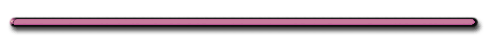AP Physics 1Distance and Motion
St. Mary's HSC. Acceleration - time rate of change in velocity (how quickly velocity is changing)

Which car has the greater acceleration?

 t(sec) t = 0 t = 1 t = 2 t = 3 t = 4 t = 5 Car A 70 m/s 72 m/s 74 m/s 76 m/s 78 m/s 80 m/s Car B 0 m/s 3 m/s 6 m/s 9 m/s 12 m/s 15 m/s Car C 90 m/s 90 m/s 90 m/s 90 m/s 90 m/s 90 m/s Car D 100 m/s 99 m/s 98 m/s 97 m/s 96 m/s 95 m/s

Car A

+2 m/s/s

Car B

+3 m/s/s

Car C

0 m/s/s

Car D

- 1 m/s/s

 t(sec) t = 0 t = 1 t = 2 t = 3 t = 4 t = 5 Car A 70 m/s 72 m/s 74 m/s 76 m/s 78 m/s 80 m/s Car B 0 m/s 3 m/s 6 m/s 9 m/s 12 m/s 15 m/s Car C 90 m/s 90 m/s 90 m/s 90 m/s 90 m/s 90 m/s Car D 100 m/s 99 m/s 98 m/s 97 m/s 96 m/s 95 m/s

2 Ways to Have
Zero Acceleration

a) Remain at Restb) Constant Velocity1. Equation

a = ∆V/∆t  = (Vf - Vi)/t

Ex 1Starting Velocity: 80 km/h West

Final Velocity: 110 km/h West

Acceleration:  1.6 m/s2

How long did it accelerate?

(Vf - Vi) = 30 km/h W

Convert to m/s2

30 km/hrx[1000m/km]x[1 h/3600 sec]

= 8 m/s

a = (Vf - Vi)/t

1.6 m/s2 = (8 m/s)/t

t = 5 sec

An object has an
acceleration of
-12 m/s2

Is it accelerating or decelerating?

Depends ....

If velocity is negative, accelerating

ex) -100 m/s to -124 m/s in 2 sec

If velocity is +, decelerating

124 m/s to 100 m/s in 2 sec

 Acceleration V +,  A+ V-, A- Deceleration V+, A- V-, A+'Accelerating Backwards'

(Because the force required to slow an object
always opposes the objects motion)